# Creating a map with contour lines¶

Plotting a contour map is handled by `pygmt.Figure.grdcontour`.

```import pygmt

# Load sample earth relief data
grid = pygmt.datasets.load_earth_relief(resolution="05m", region=[-92.5, -82.5, -3, 7])
```

## Create contour plot¶

The `pygmt.Figure.grdcontour` method takes the grid input. It plots annotated contour lines, which are thicker and have the elevation/depth written on them, and unannotated contour lines. In the example below, the default contour line intervals are 500 meters, with an annotated contour line every 1000 meters. By default, it plots the map with the equidistant cylindrical projection and with no frame.

```fig = pygmt.Figure()
fig.grdcontour(grid=grid)
fig.show()
```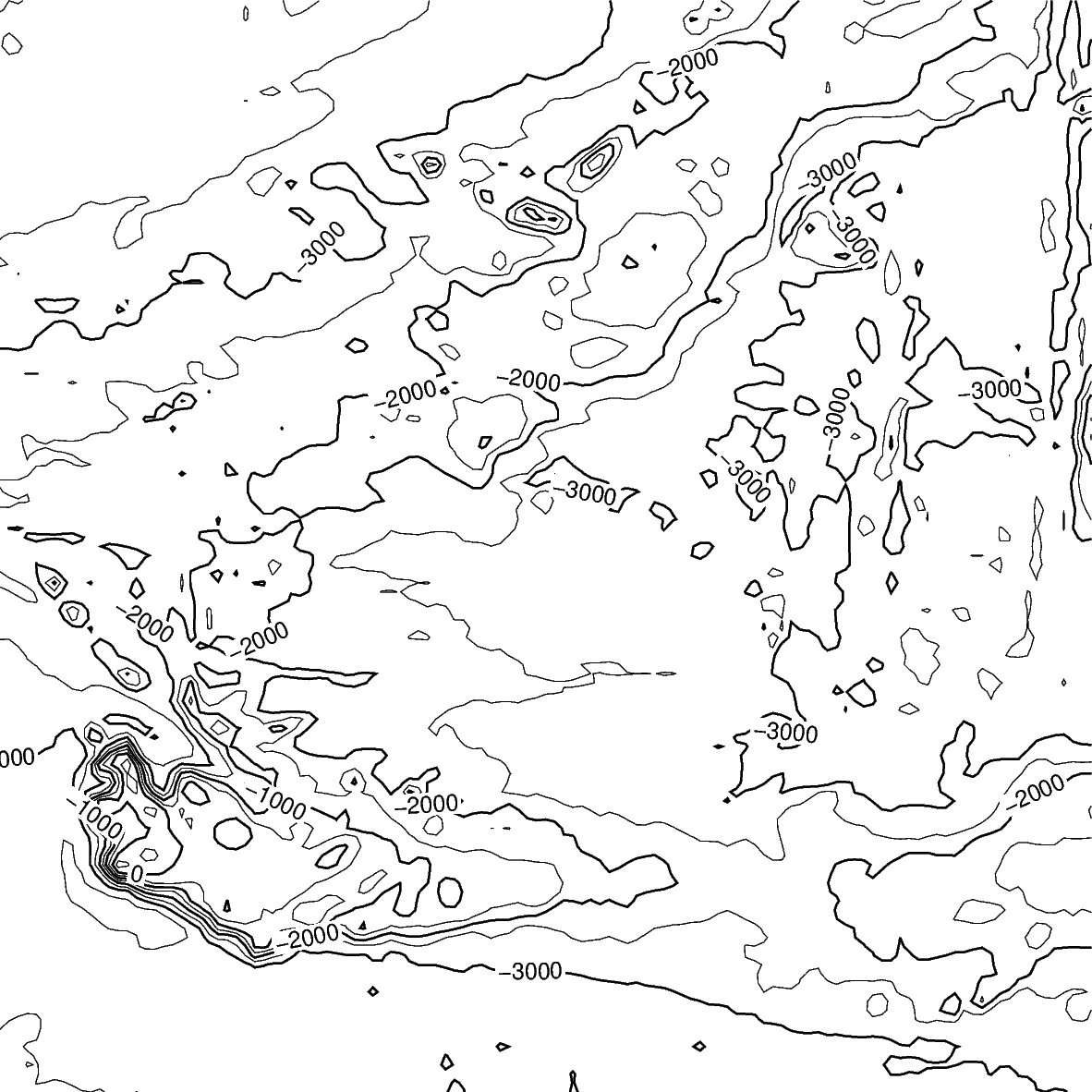Out:

```<IPython.core.display.Image object>
```

## Contour line settings¶

Use the `annotation` and `interval` arguments to adjust contour line intervals. In the example below, there are contour intervals every 250 meters and annotated contour lines every 1,000 meters.

```fig = pygmt.Figure()
fig.grdcontour(
annotation=1000,
interval=250,
grid=grid,
)
fig.show()
```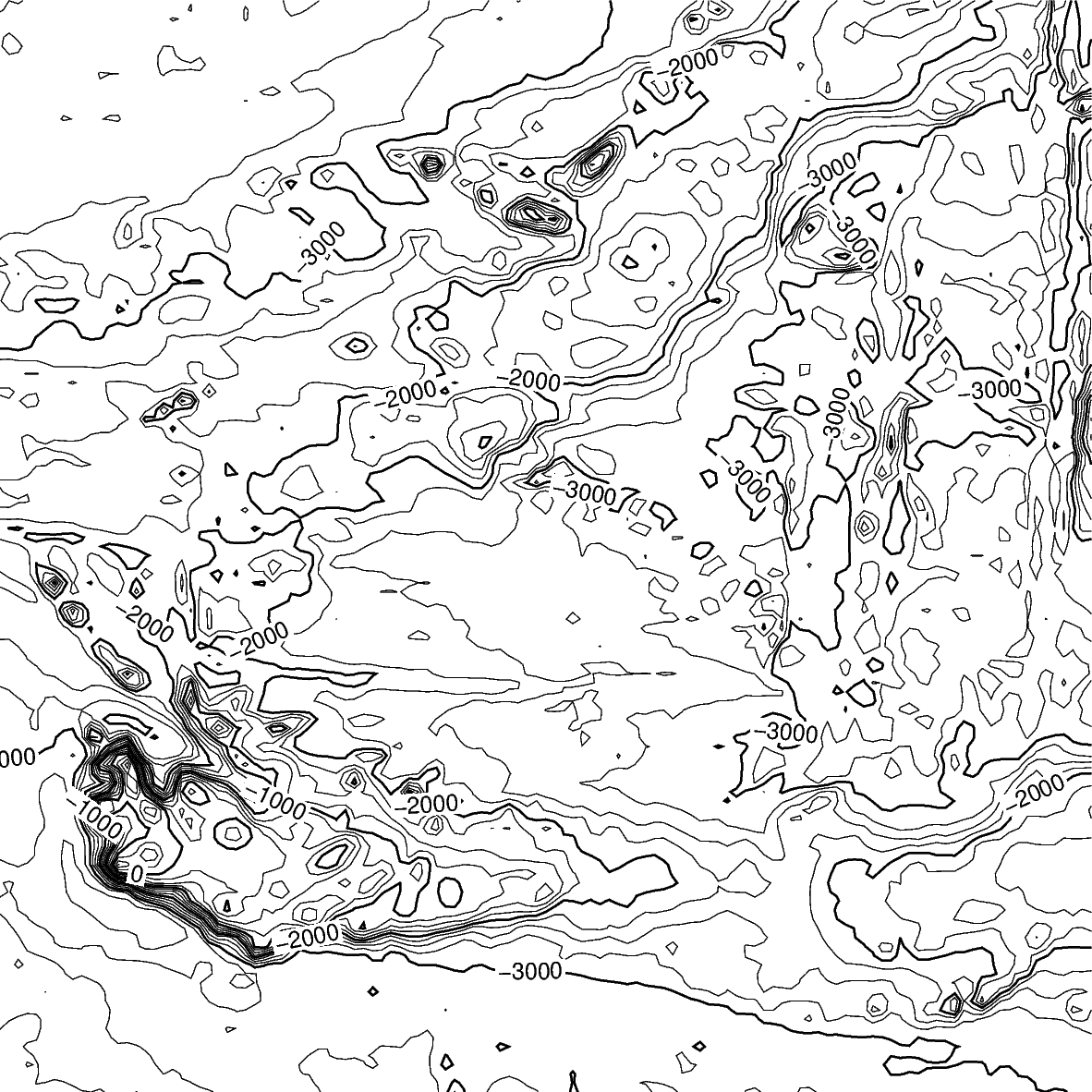Out:

```<IPython.core.display.Image object>
```

## Contour limits¶

The `limit` argument sets the minimum and maximum values for the contour lines. The argument takes the low and high values, and is either a list (as below) or a string `limit="-4000/-2000"`.

```fig = pygmt.Figure()
fig.grdcontour(
annotation=1000,
interval=250,
grid=grid,
limit=[-4000, -2000],
)
fig.show()
```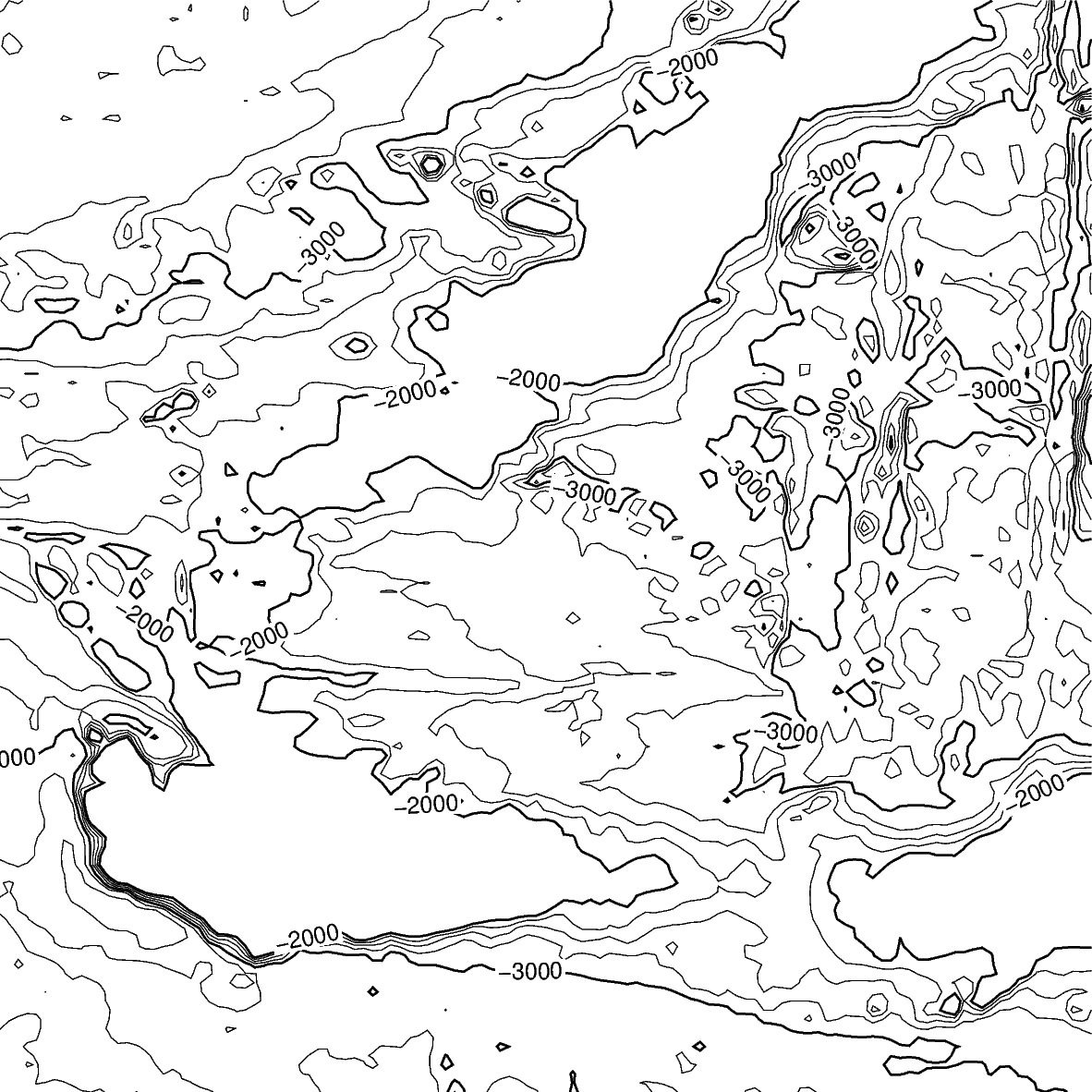Out:

```<IPython.core.display.Image object>
```

## Map settings¶

The `pygmt.Figure.grdcontour` method accepts additional arguments, including setting the projection and frame.

```fig = pygmt.Figure()
fig.grdcontour(
annotation=1000,
interval=250,
grid=grid,
limit=[-4000, -2000],
projection="M10c",
frame=True,
)
fig.show()
```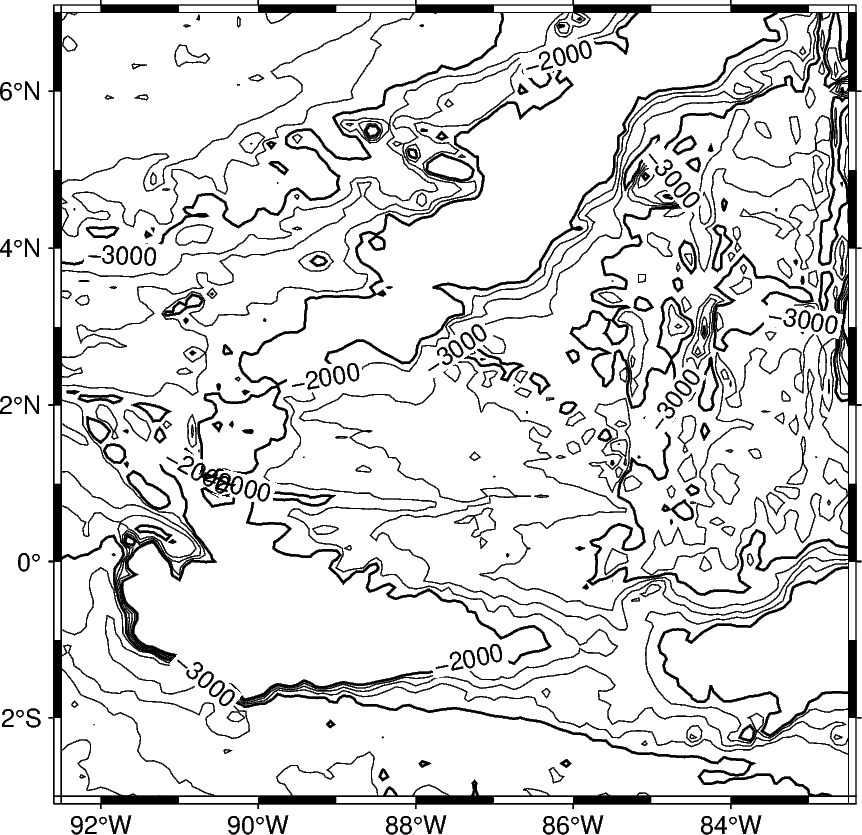Out:

```<IPython.core.display.Image object>
```

## Adding a colormap¶

The `pygmt.Figure.grdimage` method can be used to add a colormap to the contour map. It must be called prior to `pygmt.Figure.grdcontour` to keep the contour lines visible on the final map. If the `projection` argument is specified in the `pygmt.Figure.grdimage` method, it does not need to be repeated in the `pygmt.Figure.grdcontour` method.

```fig = pygmt.Figure()
fig.grdimage(
grid=grid,
cmap="haxby",
projection="M10c",
frame=True,
)
fig.grdcontour(
annotation=1000,
interval=250,
grid=grid,
limit=[-4000, -2000],
)
fig.show()
```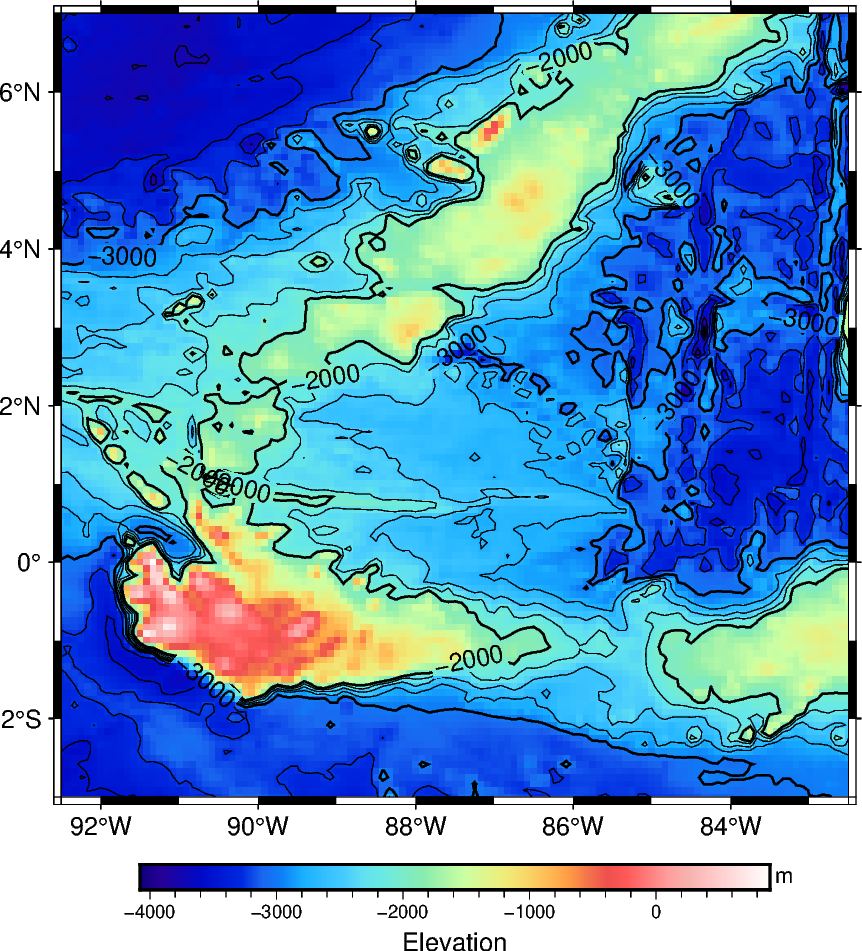Out:

```<IPython.core.display.Image object>
```

Total running time of the script: ( 0 minutes 7.553 seconds)

Gallery generated by Sphinx-Gallery